# Lecture Power Points Chapter 20 Physics Principles with

• Slides: 26Lecture Power. Points Chapter 20 Physics: Principles with Applications, 6 th edition Giancoli © 2005 Pearson Prentice Hall This work is protected by United States copyright laws and is provided solely for the use of instructors in teaching their courses and assessing student learning. Dissemination or sale of any part of this work (including on the World Wide Web) will destroy the integrity of the work and is not permitted. The work and materials from it should never be made available to students except by instructors using the accompanying text in their classes. All recipients of this work are expected to abide by these restrictions and to honor the intended pedagogical purposes and the needs of other instructors who rely on these materials.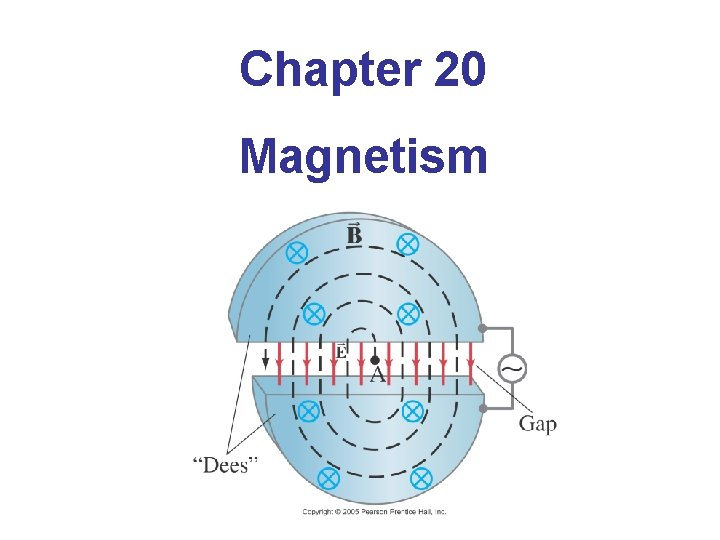Chapter 20 Magnetism20. 1 Magnets and Magnetic Fields Magnets have two ends – poles – called north and south. Like poles repel; unlike poles attract.20. 1 Magnets and Magnetic Fields However, if you cut a magnet in half, you don’t get a north pole and a south pole – you get two smaller magnets.20. 1 Magnets and Magnetic Fields Magnetic fields can be visualized using magnetic field lines, which are always closed loops. Notice that the magnetic field goes from North to South.20. 1 Magnets and Magnetic Fields The Earth’s magnetic field is similar to that of a bar magnet. Note that the Earth’s “North Pole” is really a south magnetic pole, as the north ends of magnets are attracted to it.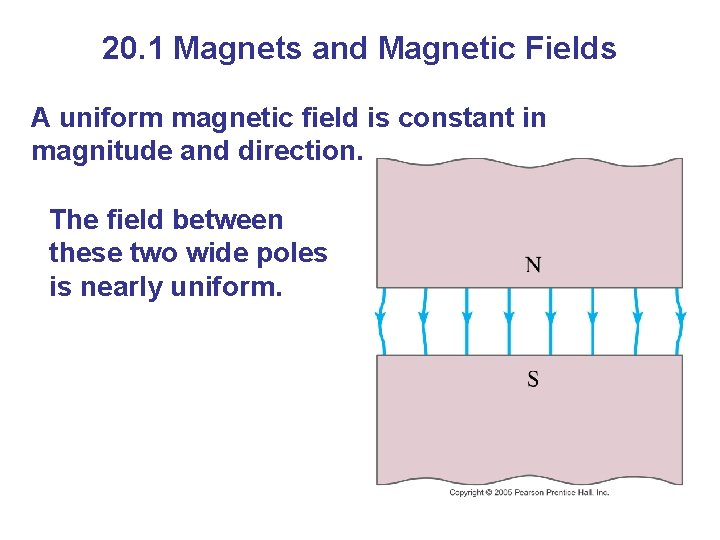20. 1 Magnets and Magnetic Fields A uniform magnetic field is constant in magnitude and direction. The field between these two wide poles is nearly uniform.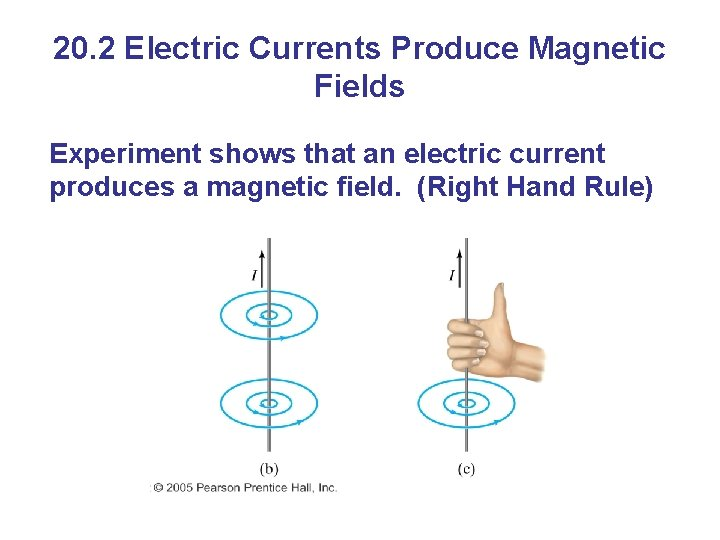20. 2 Electric Currents Produce Magnetic Fields Experiment shows that an electric current produces a magnetic field. (Right Hand Rule)Magnetic field lines due to a circular loop of wire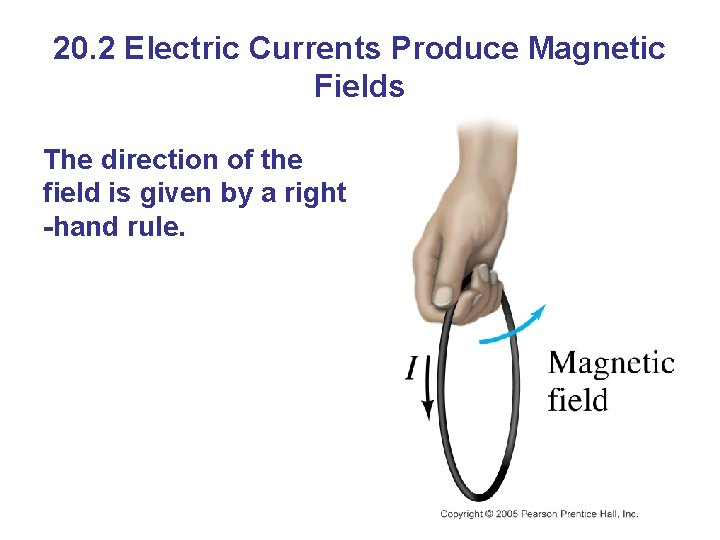20. 2 Electric Currents Produce Magnetic Fields The direction of the field is given by a right -hand rule.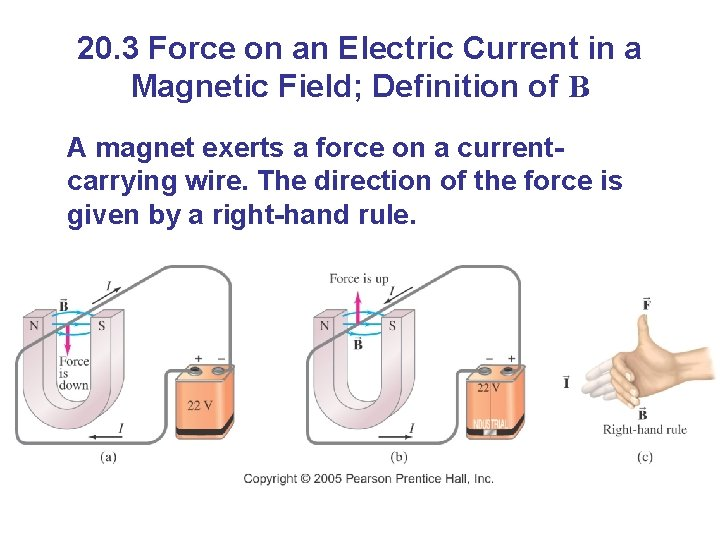20. 3 Force on an Electric Current in a Magnetic Field; Definition of B A magnet exerts a force on a currentcarrying wire. The direction of the force is given by a right-hand rule.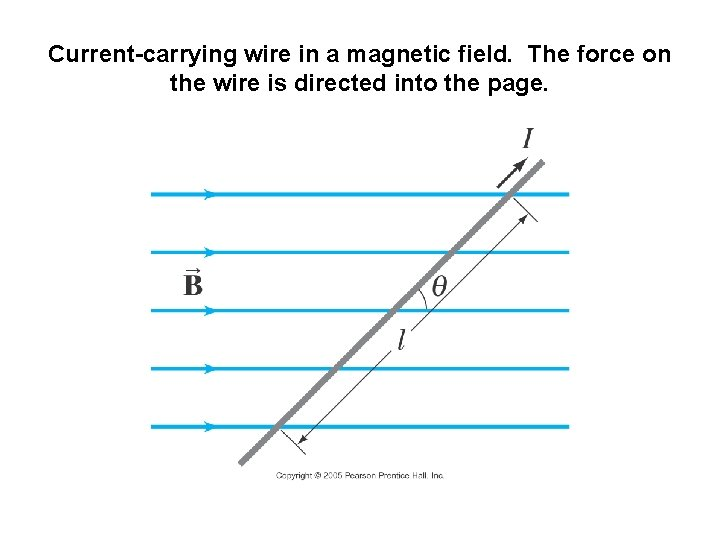Current-carrying wire in a magnetic field. The force on the wire is directed into the page.20. 3 Force on an Electric Current in a Magnetic Field; Definition of B The force on the wire depends on the current, the length of the wire, the magnetic field, and its orientation. (on formula sheet) If the direction of I is perpendicular to B, then θ = 90˚ and sin θ = 1. If I is parallel to B, then θ = 0˚ and sin θ = 0. This equation defines the magnetic field B.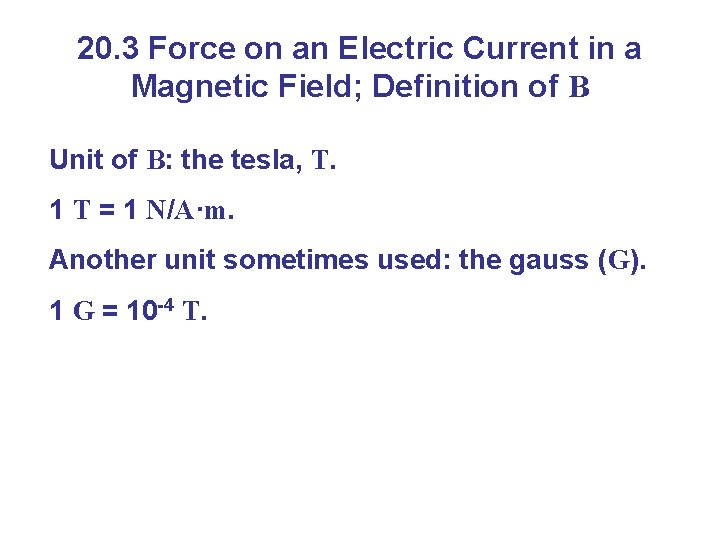20. 3 Force on an Electric Current in a Magnetic Field; Definition of B Unit of B: the tesla, T. 1 T = 1 N/A·m. Another unit sometimes used: the gauss (G). 1 G = 10 -4 T.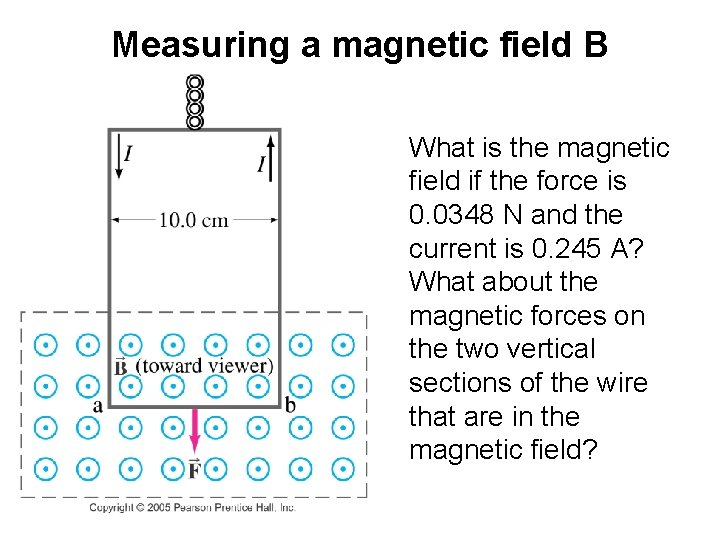Measuring a magnetic field B What is the magnetic field if the force is 0. 0348 N and the current is 0. 245 A? What about the magnetic forces on the two vertical sections of the wire that are in the magnetic field?20. 4 Force on Electric Charge Moving in a Magnetic Field The force on a moving charge is related to the force on a current: (on formula sheet) The force is greatest when the particle moves perpendicular to B (θ = 90°) Once again, the direction is given by a right-hand rule. The rule is for positive particles. Notice the difference between positive and negative particles.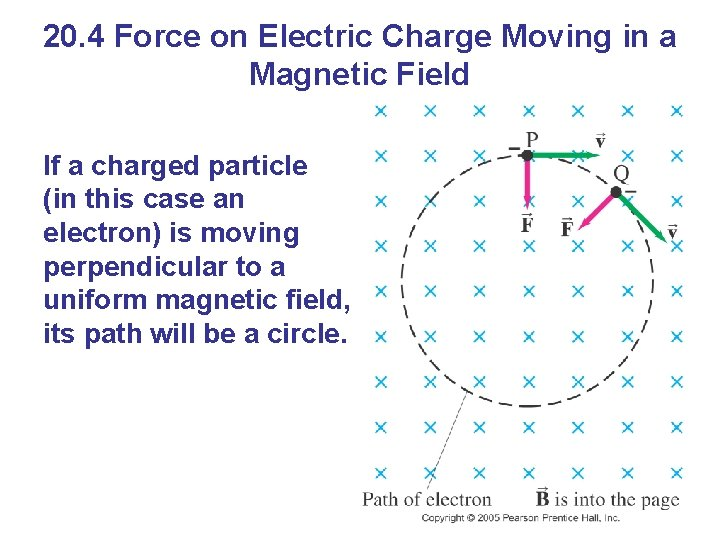20. 4 Force on Electric Charge Moving in a Magnetic Field If a charged particle (in this case an electron) is moving perpendicular to a uniform magnetic field, its path will be a circle.What is the path of a charged particle if its velocity is not perpendicular to the magnetic field? The parallel component of v experiences no force, so it remains constant. The perpendicular component of v results in circular motion. Together this produces a spiral motion.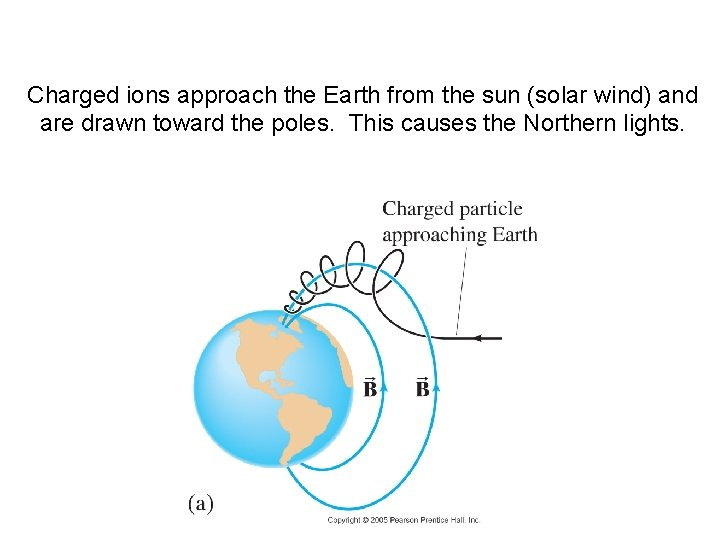Charged ions approach the Earth from the sun (solar wind) and are drawn toward the poles. This causes the Northern lights.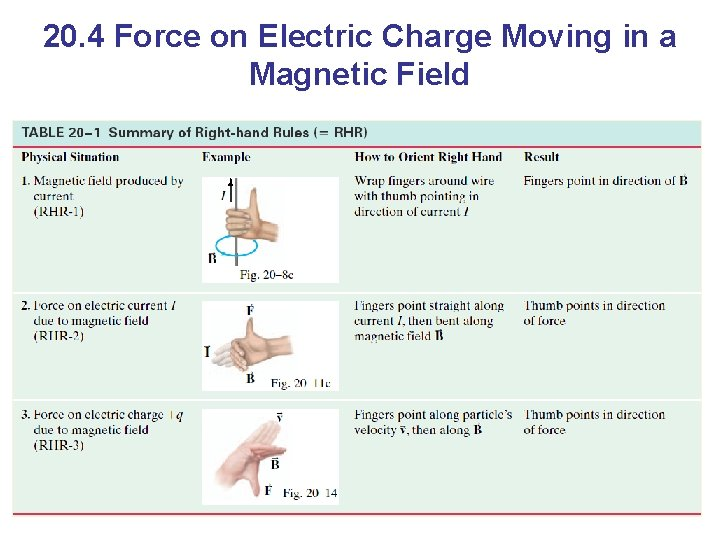20. 4 Force on Electric Charge Moving in a Magnetic Field20. 5 Magnetic Field Due to a Long Straight Wire The field is inversely proportional to the distance from the wire: (on formula sheet) The constant μ 0 is called the permeability of free space (or Vacuum permeability), and has the value:20. 6 Force between Two Parallel Wires The magnetic field produced at the position of wire 2 due to the current in wire 1 is: The force this field exerts on a length l 2 of wire 2 is: (20 -6)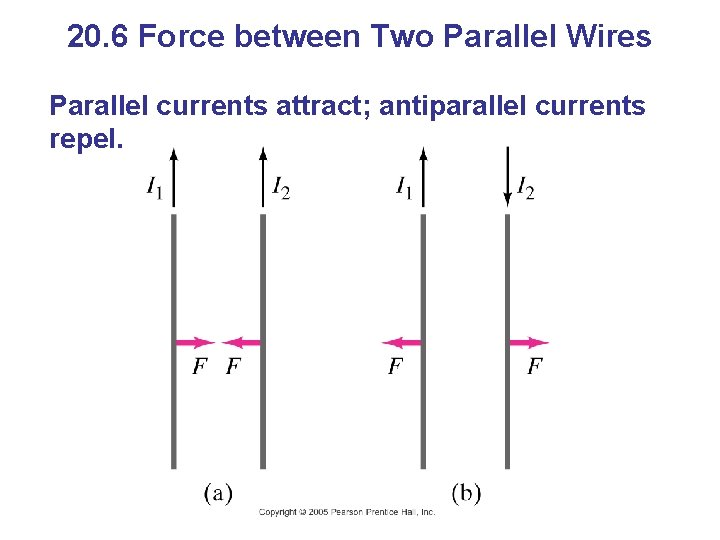20. 6 Force between Two Parallel Wires Parallel currents attract; antiparallel currents repel.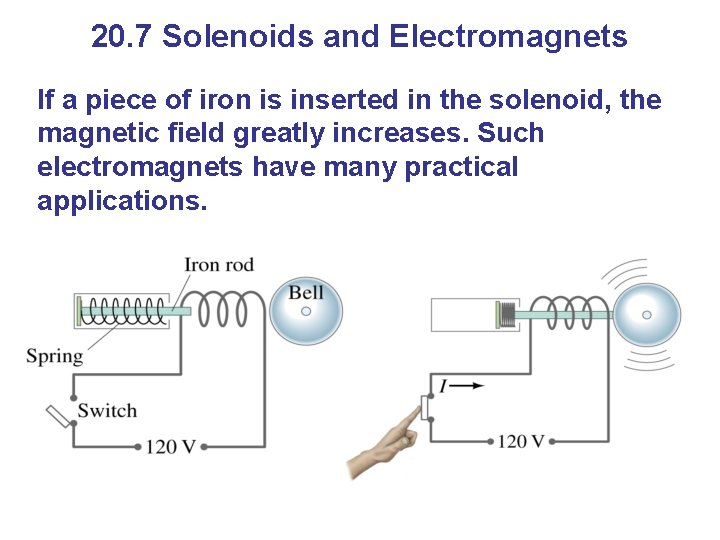20. 7 Solenoids and Electromagnets If a piece of iron is inserted in the solenoid, the magnetic field greatly increases. Such electromagnets have many practical applications.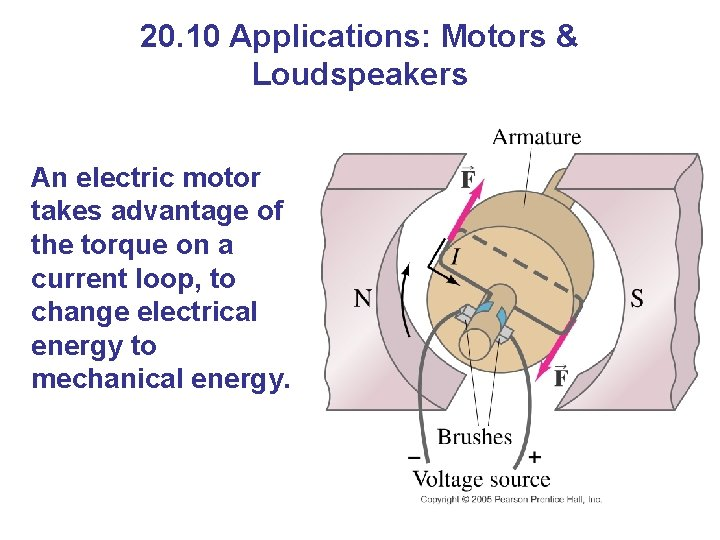20. 10 Applications: Motors & Loudspeakers An electric motor takes advantage of the torque on a current loop, to change electrical energy to mechanical energy.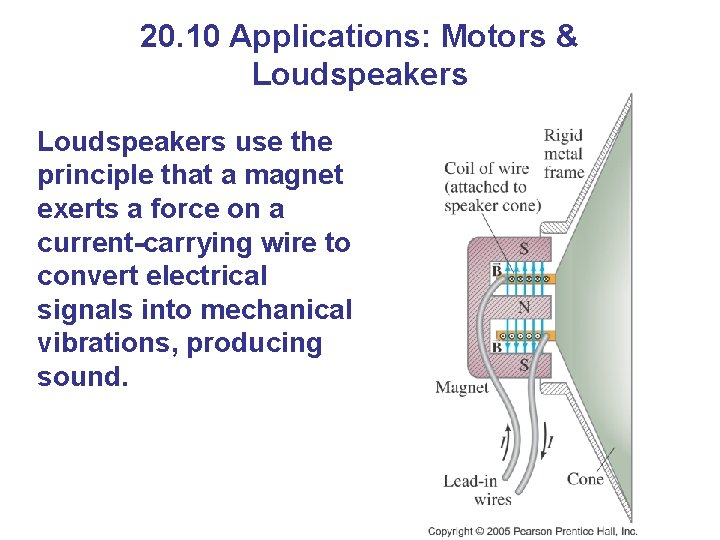20. 10 Applications: Motors & Loudspeakers use the principle that a magnet exerts a force on a current-carrying wire to convert electrical signals into mechanical vibrations, producing sound.